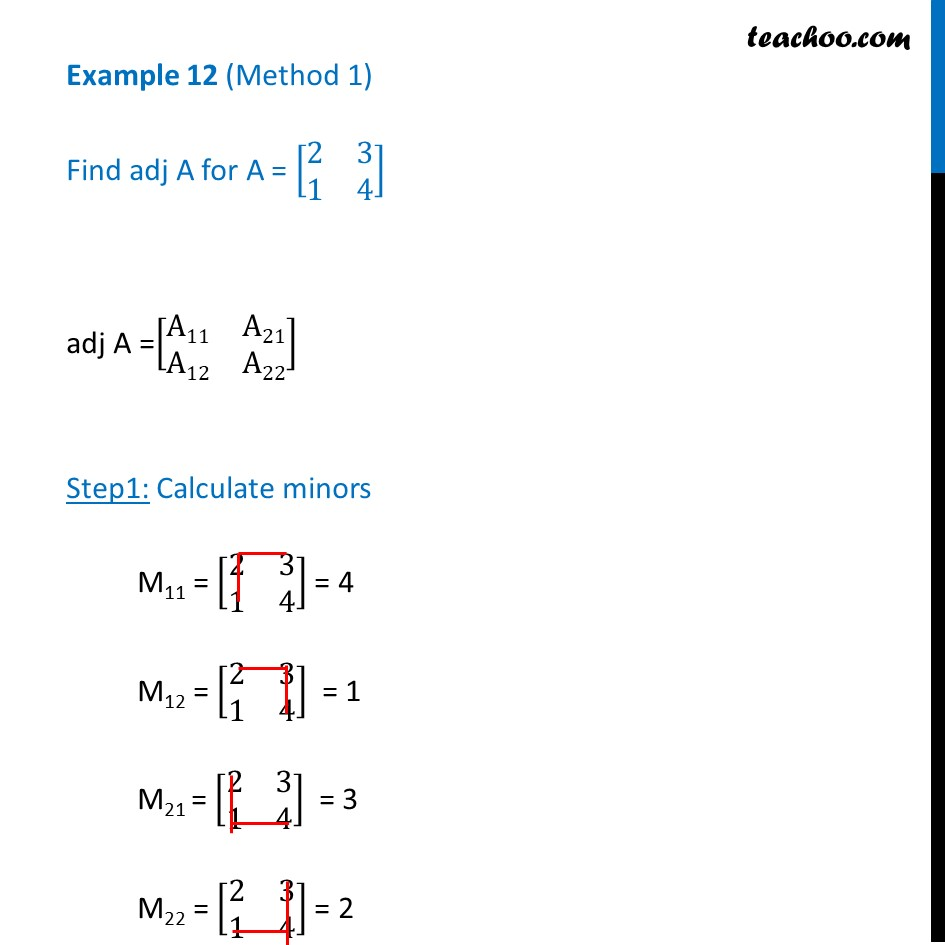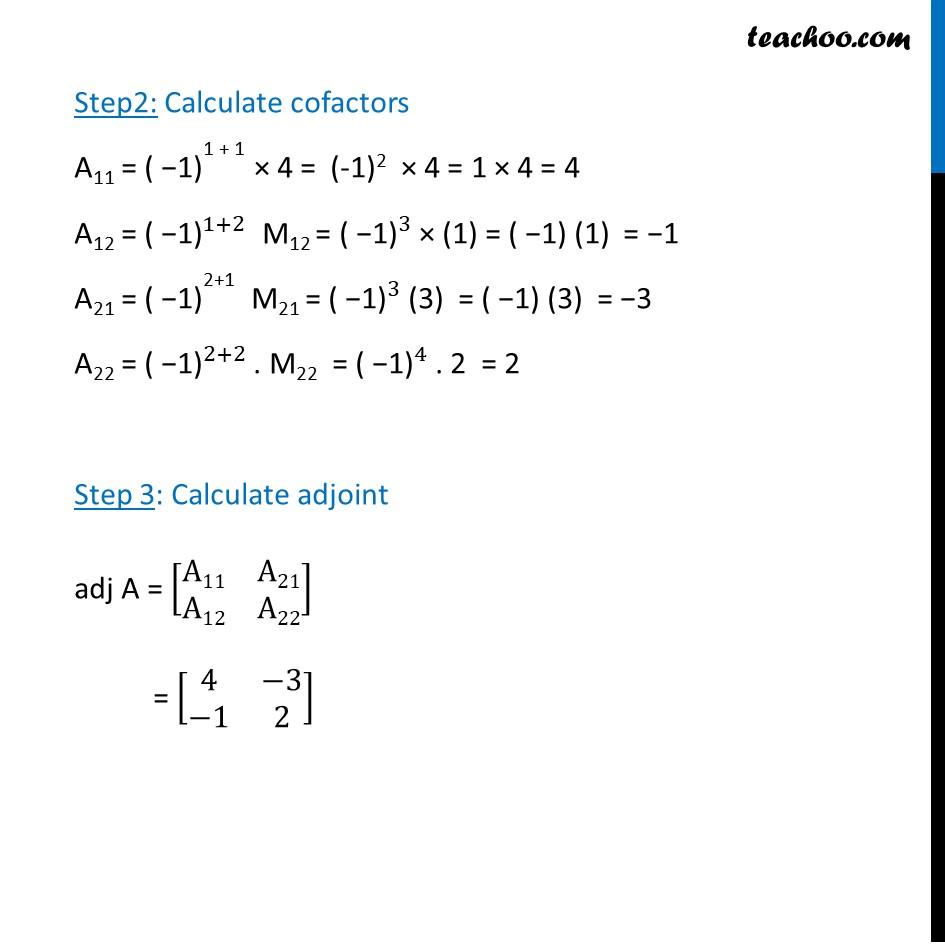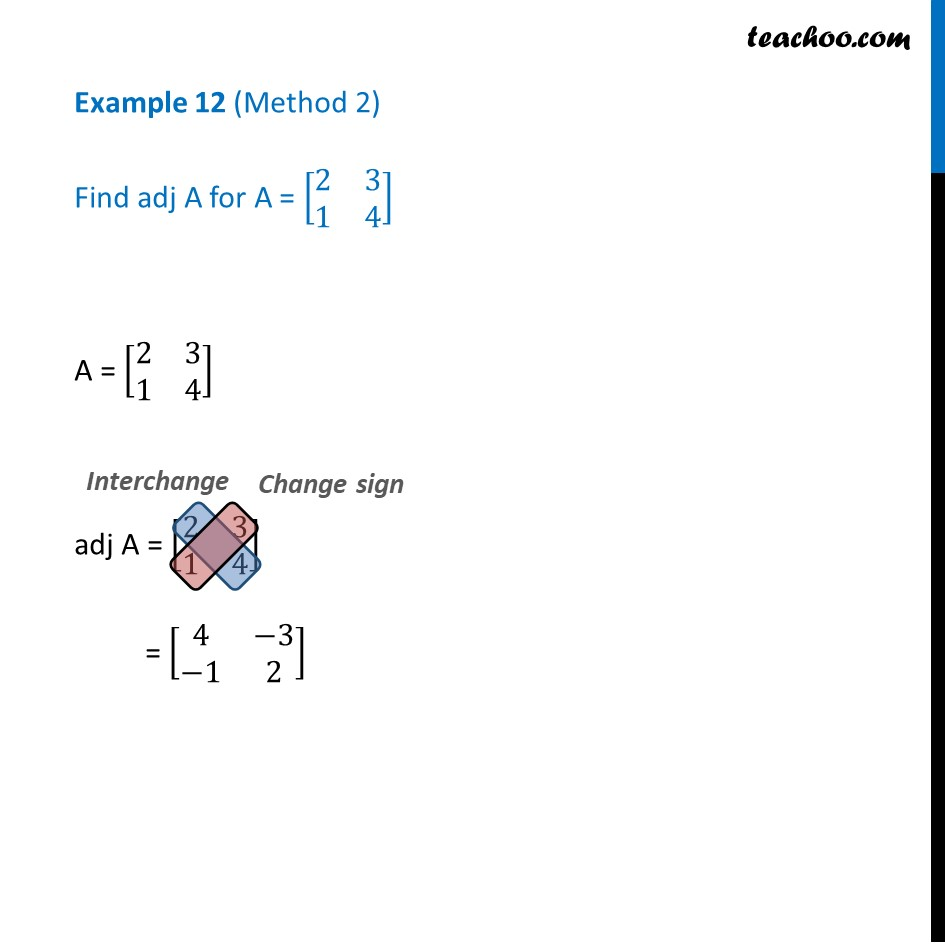Examples

Chapter 4 Class 12 Determinants
Serial order wiseLearn in your speed, with individual attention - Teachoo Maths 1-on-1 Class

### Transcript

Example 12 (Method 1) Find adj A for A = [■8(2&3@1&4)] adj A =[■8(A11&A21@A12&A22)] Step1: Calculate minors M11 = [■8(2&3@1&4)] = 4 M12 = [■8(2&3@1&4)] = 1 M21 = [■8(2&3@1&4)] = 3 M22 = [■8(2&3@1&4)] = 2 Step2: Calculate cofactors A11 = 〖"( −1)" 〗^"1 + 1" × 4 = (-1)2 × 4 = 1 × 4 = 4 A12 = 〖"( −1)" 〗^(1+2) M12 = 〖"( −1)" 〗^3 × (1) = ( −1) (1) = −1 A21 = 〖"( −1)" 〗^"2+1" M21 = 〖"( −1)" 〗^3 (3) = ( −1) (3) = −3 A22 = 〖"( −1)" 〗^(2+2) . M22 = 〖"( −1)" 〗^4 . 2 = 2 Step 3: Calculate adjoint adj A = [■8(A11&A21@A12&A22)] = [■8(4&−3@−1&2)] Example 12 (Method 2) Find adj A for A = [■8(2&3@1&4)] A = [■8(2&3@1&4)] adj A = [■8(2&3@1&4)] = [■8(4&−3@−1&2)]